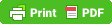# The Functional Approach Part 2

Hello, and welcome to part 2 of approaching some programming problems with functional methodologies. In the last post, I had concentrated on two key areas: Transforming one list into another with the application of an algorithm on each original item to obtain the transformed item, and Filtering the original list based on a predicate to obtain a list with possibly less items than the original.

Today I will discuss the third aspect of functional list processing: Folding.

Folding a list yields exactly one final value. That value depends on the items in the list, and a function that takes the first element of the list, and a start value. The returned result is then used as the “start value” for the next iteration of the fold. In this next iteration the first element of the list is popped off, and the next element becomes the first (we have a shorter list). This process goes on until the entire list is exhausted and the final result is the start value that would have been used had the list had more elements.

Example:

var list = [1, 2, 3]; alert(fold(list, 0, function(start, item) { return start + item; }));

Here, we see a list with values 1, 2, and 3. In the first iteration the start value, 0 in this case, is used as the first parameter to the supplied function and 1, the first element of the list is the second argument. The function then returns their sum (1) to be used as the start argument to the same function in the next iteration. This time however the item parameter will take on the value 2, and the function will sum them up to yield the result 3, which again becomes the start value for the next iteration of the function call. The second parameter is 3 (the last item of our list). The final sum then becomes 6, and since there are no more items, that is the final value of the call to fold. So the alert box pops up with the value of 6.

Final thoughts: By combining the transform, filter and fold algorithms together to use and manipulate lists of items, a section of code can become very condensed and localized, reduced in complexity, and become self documenting. Like with all tools, there is a risk of misuse. My suggestion in this regard would be to first figure out whether a piece of code lends itself to this pattern. A lot of the time list processing code fits the bill quite well. However, this is not a magic bullet. If the occasion calls for an object with state and methods, then by all means write a class or a prototype!http://blogs.citydirect.info/developing-web-apps/?p=87#comment-